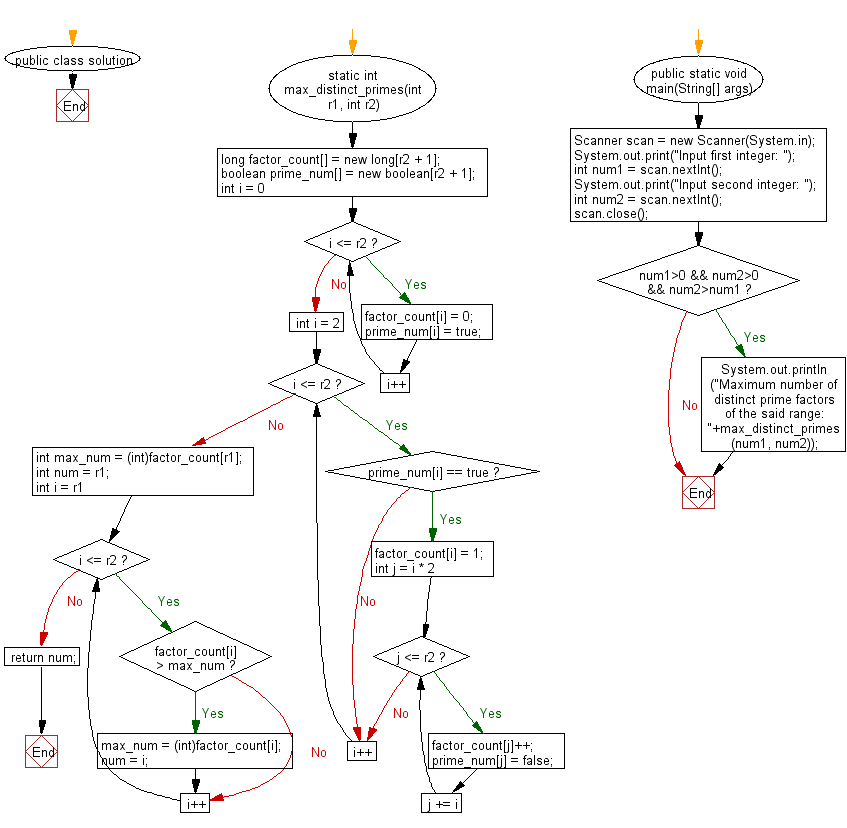﻿ Java Math Exercises: Find the number which has the maximum number of distinct prime factors in a given range - w3resource# Java Math Exercises: Find the number which has the maximum number of distinct prime factors in a given range

## Java Math Exercises: Exercise-21 with Solution

Write a Java program to find the number which has the maximum number of distinct prime factors in a given range.

Sample Solution:

Java Code:

``````import java.util.*;

public class solution {
static int max_distinct_primes(int r1, int r2)
{
long factor_count[] = new long[r2 + 1];
boolean prime_num[] = new boolean[r2 + 1];
for (int i = 0; i <= r2; i++)
{
factor_count[i] = 0;
prime_num[i] = true;
}

for (int i = 2; i <= r2; i++)
{
if (prime_num[i] == true)
{

factor_count[i] = 1;
for (int j = i * 2; j <= r2; j += i)
{
factor_count[j]++;
prime_num[j] = false;
}
}
}

int max_num = (int)factor_count[r1];
int num = r1;

for (int i = r1; i <= r2; i++)
{
if (factor_count[i] > max_num)
{
max_num = (int)factor_count[i];
num = i;
}
}
return num;
}

public static void main(String[] args) {
Scanner scan = new Scanner(System.in);
System.out.print("Input first integer: ");
int num1 = scan.nextInt();
System.out.print("Input second integer: ");
int num2 = scan.nextInt();
scan.close();
if (num1>0 && num2>0 && num2>num1)
{
System.out.println("Maximum number of distinct prime factors of the said range: "+max_distinct_primes(num1, num2));
}
}
}
``````

Sample Output:

```Input first integer:  5
Input second integer:  25
Maximum number of distinct prime factors of the said range: 6
```

Flowchart:Java Code Editor:

What is the difficulty level of this exercise?

﻿

## Java: Tips of the Day

Parsing dates:

```import java.io.*;
import java.util.*;
import java.text.*;

String s = "2001/09/23 14:39";

SimpleDateFormat formatter = new SimpleDateFormat ("yyyy/MM/dd H:mm");
Date d = formatter.parse(s, new ParsePosition(0));
```